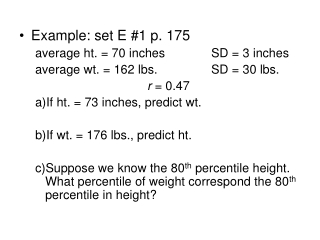# Example: set E #1 p. 175 average ht. = 70 inches SD = 3 inches - PowerPoint PPT PresentationDownload PresentationExample: set E #1 p. 175 average ht. = 70 inches SD = 3 inches

Example: set E #1 p. 175 average ht. = 70 inches SD = 3 inchesDownload Presentation## Example: set E #1 p. 175 average ht. = 70 inches SD = 3 inches

- - - - - - - - - - - - - - - - - - - - - - - - - - - E N D - - - - - - - - - - - - - - - - - - - - - - - - - - -
##### Presentation Transcript

1. Example: set E #1 p. 175 average ht. = 70 inches SD = 3 inches average wt. = 162 lbs. SD = 30 lbs. r = 0.47 • If ht. = 73 inches, predict wt. • If wt. = 176 lbs., predict ht. • Suppose we know the 80th percentile height. What percentile of weight correspond the 80th percentile in height?

2. Ch. 11 R.M.S Error for Regression • error = actual – predicted = residual • When we make a prediction we usually have some error in our prediction. • RMS(error) for regression describes how far points typically are above/below the regression line.

3. Baggage handout. y = x = • What are the cases? • What is the relationship between the 2 variables? • Is this a positive or negative association? • Average x = Average y = 5. Plots of deviations and residuals.

4. If the residual plot has a pattern to it, the linear regression was probably not well fit to the data. • The residual plot should have the points evenly spread above and below the horizontal axis.

5. Calculating RMS(error)=square root(sum of the square errors divided by the total number of values). • The RMS(error) has the same units as y (the variable being predicted. • 68% of the points should be 1 RMS(error) from the regression line • 95% of the points should be 2 RMS(error)s from the regression line • Examples (Ch.11 Set A #4, 5, 7 p. 184)

6. RMS(error) for regression line of y on x is (Use the SD of the variable being predicted.)

7. Special cases of RMS(error) for different values of r. • r = 0.3, 0.6, 0.8, 0.9, 0.95, 0.99 What happens to RMS(error) as r increases? • Homoscedasticity (football-shaped scatter diagram) • Heteroscedasticity: different scatter around the regression line • Examples #11 p. 200, p. 192 figure

8. Example (Ch.11 Set D #3 p.193) • In order to use the normal approximation, the scatter diagram should be football-shaped with points thickly scattered in the center and fading at the edges. • If a scatter diagram is football-shaped, take the points in a narrow vertical strip and they will be away from the regression line by amounts similar to the RMS(error). • The new average is estimated from the regression method • The new SD is approximately equal to the RMS(error) of the regression line. • Example set E #1 p. 197